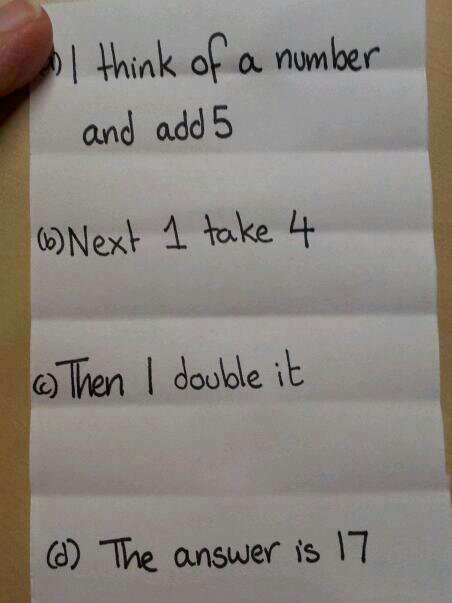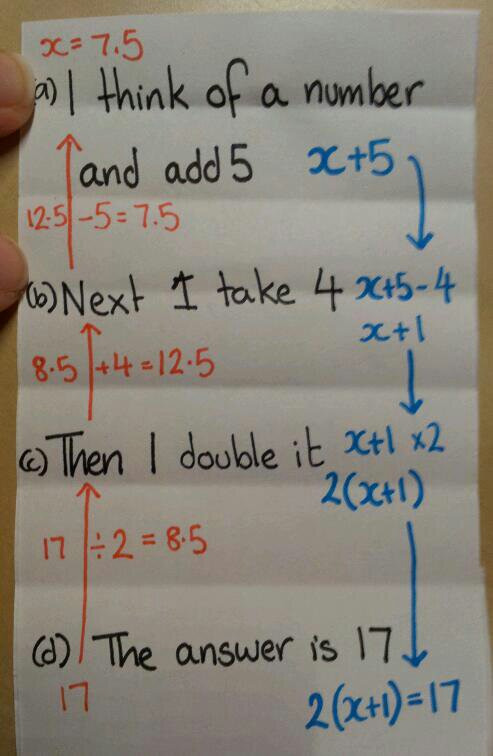# 186. Fantastical algebra

Have you ever played the parlour game ‘Fantastical Creatures’? Click for a lovely description and example of it by Little Cotton Rabbits.

I’ve adapted this concept for teaching aspects of number and algebra.

Topics
Basic arithmetic
Inverse operations
Order of operations
Setting up simple equations
Using brackets with numbers/letters
Solving single sided equations

Equipment
Strips of paper – one sheet of A4 makes about 6 strips
Coloured pens (optional)

Basic instructions
1. Write an instruction on the top of the strip (portrait orientation). Label it (a).

2. Fold over the strip twice to hide the writing. Write (b).

3. Pass on the strip, do not unfold it.
4. By the ‘(letter label)’, write the next instruction. The letters help you keep track of how many times it has been passed on.
5. Fold over the strip twice and put a label for the next letter of the alphabet.
6. Repeat steps 3 – 5 as required.

The beauty of this activity is that each problem is constructed by a group of pupils and they are in control of the level of difficulty.

Activity 1: Setting up simple equations

Follow the basic activities with the following instructions:
(a) I think of a number and write an instruction
(b) & (c) Now I write an instruction
(d) The answer is write a numberPupils fold the puzzle up tight and either pass it on one last time or hand them in (to be randomly distributed).

Pupils unfold their mystery puzzle and construct the equation, step by step. My pupils quickly realised the importance of simplifying, but many forgot the importance of using brackets. This was a useful misconception to identify.

Pupils then use inverse operations to calculate the unknown.The algebraic operations and numerical operations can then be compared.

Activity 2: Problem solving

This follows the same structure as the equation activity, but pupils are describing a geometric problem. In the examples the blue sections are up to the pupils to choose.

Example 2

In the second example pupils can visualise the problem as well as using algebraic terms.

Activity 3: Number Skills

This activity can also be used for setting up BIDMAS problems by omitting the algebra.